# Directional coupler¶

SiFab contains five different directional couplers:

• DirectionalCoupler: fully parametric directional coupler.
• DirectionalCouplerU: directional coupler with a U-shape. It has fixed properties, but variable length of the straight section.
• DirectionalCouplerS: directional coupler with an S-shape. It has fixed properties, but variable length of the straight section.
• DirectionalCouplerUPower: directional coupler with a U-shape. The desired power split ratio between the two arms can be specified and the correct length is automatically calculated.
• DirectionalCouplerSPower: directional coupler with an S-shape. The desired power split ratio between the two arms can be specified and the correct length is automatically calculated.

The section Simulation and regeneration of the data files explains how to simulate and regenerate the simulation data of these directional couplers.

## DirectionalCoupler¶

This is a directional coupler with fully customisable layout. Custom functions can be passed as routing_method. This function is used to draw the bent section of the directional coupler and takes three parameters as input: bend_width, bend_height and adiabatic_angle. The currently available routing methods are called ShapeDCBend, to obtain a U-shaped directional coupler, and ShapeDCSBend, to obtain an S-shaped directional coupler. This class doesn’t have a circuit model as the directional coupler has not been simulated and optimized.

Reference

Click on the name of the component below to see the complete PCell reference.

 si_fab.all.DirectionalCoupler Directional coupler with customisable layout.

Click on the name of the functions below to see the complete API reference of the two available routing methods.

 si_fab.components.dir_coupler.pcell.cell_utils.ShapeDCBend Method that returns a Bezier bend to be used to build the directional coupler. si_fab.components.dir_coupler.pcell.cell_utils.ShapeDCSBend Method that returns a Bezier s-bend to be used to build the directional coupler.

Example

from si_fab import all as pdk
from si_fab.components.dir_coupler.pcell.cell_utils import ShapeDCSBend, ShapeDCBend

dc1 = pdk.DirectionalCoupler(routing_method=ShapeDCSBend,
straight_length=5.0,
spacing=0.1,
bend_length=5.0,
bend_height=1.0,

dc1_lv = dc1.Layout()
dc1_lv.visualize(annotate=True)

dc2 = pdk.DirectionalCoupler(routing_method=ShapeDCBend,
straight_length=5.0,
spacing=0.1,
bend_length=4.0,

dc2_lv = dc2.Layout()
dc2_lv.visualize(annotate=True)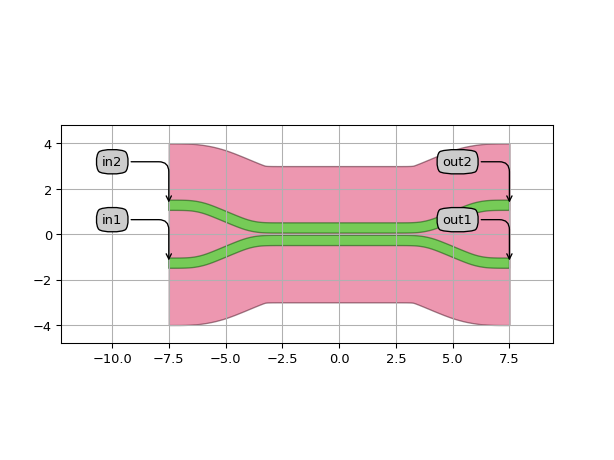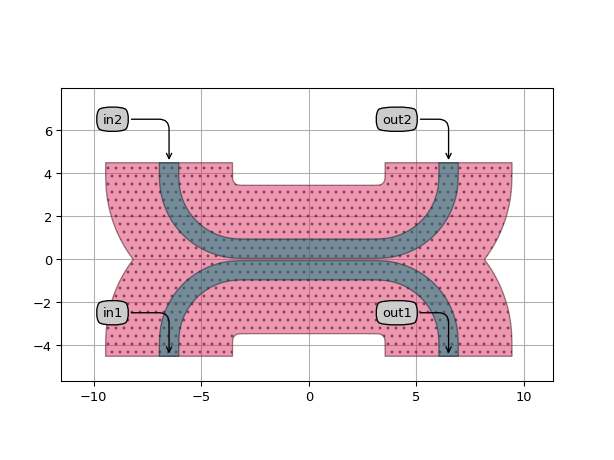## DirectionalCouplerU¶

This directional coupler inherits from DirectionalCoupler and uses a Bezier bend as standard routing method for the bent section. All the properties, aside from straight_length, are locked and have been optimized and simulated in the wavelength range between 1500 nm and 1600 nm using Ansys Lumerical FDTD and CST Studio Suite ®.

After the simulation, a fitting of the transmission and of the coupling parameters ($$\kappa$$ and $$\kappa _0$$) is performed as a function of length for all the wavelengths between 1500 nm and 1600 nm. The coefficients of the polynomial fit are stored and used by the circuit model of this component to run Caphe simulations with the desired length and at the desired wavelength. For this component, an analytical model with the following equations is used:

$\begin{split}\begin{array}{c} S[in_1, out_1] &=& S[out_1, in_1] &=& \text{through} &=& t \sin(\kappa_0 + \kappa*L)\\ S[in_1, out_2] &=& S[out_1, in_1] &=& \text{cross} &=& 1\jmath t \cos(\kappa_0 + \kappa*L) \end{array}\end{split}$

where $$t$$ is the transmission, $$\kappa_0$$ is the coupling for a directional coupler with $$L=0$$, $$\kappa$$ is the coupling constant per unit of length. $$t$$, $$\kappa_0$$, $$\kappa$$ are dispersive with respect to wavelength.

By default, the results of the fitting of the Ansys Lumerical FDTD simulation are stored and used for the circuit simulation. However, this can be changed by regenerating the fit using the simulation data from the CST Studio Suite ® simulations (see Simulation and regeneration of the data files:).

Reference

Click on the name of the component below to see the complete PCell reference.

 si_fab.all.DirectionalCouplerU Directional coupler with a U-shape, where all the properties aside from the length (straight_length) are optimized and locked.

Example

from si_fab import all as pdk
import numpy as np
import pylab as plt

dc = pdk.DirectionalCouplerU(straight_length=8.0)
dc_lv = dc.Layout()
dc_lv.visualize(annotate=True)

dc_cm = dc.CircuitModel()

wavelengths = np.linspace(1.51, 1.6, 500)
S = dc_cm.get_smatrix(wavelengths=wavelengths)
plt.figure()
plt.plot(wavelengths, np.abs(S["in1", "out1"])**2, linewidth=2.2, label="in1-out1 (through)")
plt.plot(wavelengths, np.abs(S["in1", "out2"])**2, linewidth=2.2, label="in1-out2 (drop)")
plt.title("Power transmission", fontsize=16)
plt.xlabel("Wavelength [um]", fontsize=16)
plt.ylabel("Power [dB]", fontsize=16)
plt.xlim(1.5, 1.6)
plt.legend(fontsize=14, loc=1)
plt.show()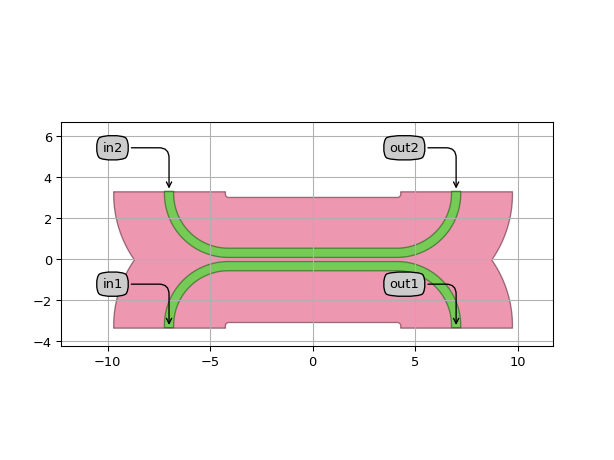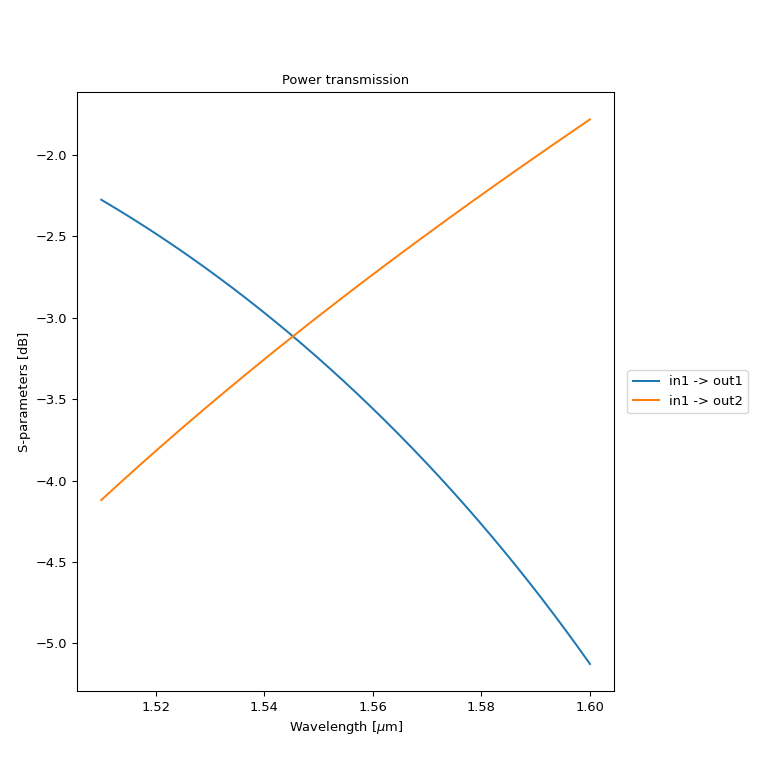## DirectionalCouplerS¶

This directional coupler follows the same logic as DirectionalCouplerU. It inherits from DirectionalCoupler and uses a Bezier s-bend as standard routing method for the bent section. All the properties, aside from straight_length, are locked and have been optimized and simulated in the wavelength range between 1500 nm and 1600 nm using Ansys Lumerical FDTD and CST Studio Suite ®. By default, the results of the fitting of the Ansys Lumerical FDTD simulation are stored and used for the circuit simulation. However, this can be changed by regenerating the fit using the simulation data from the CST Studio Suite ® simulations (see Simulation and regeneration of the data files:).

Reference

Click on the name of the component below to see the complete PCell reference.

 si_fab.all.DirectionalCouplerS Directional coupler with a S-shape, where all the properties aside from the length (straight_length) are optimized and locked.

Example

from si_fab import all as pdk
import numpy as np
import pylab as plt

dc = pdk.DirectionalCouplerS(straight_length=10.0)
dc_lv = dc.Layout()
dc_lv.visualize(annotate=True)

dc_cm = dc.CircuitModel()

wavelengths = np.linspace(1.51, 1.6, 500)
S = dc_cm.get_smatrix(wavelengths=wavelengths)
plt.figure()
plt.plot(wavelengths, np.abs(S["in1", "out1"])**2, linewidth=2.2, label="in1-out1 (through)")
plt.plot(wavelengths, np.abs(S["in1", "out2"])**2, linewidth=2.2, label="in1-out2 (drop)")
plt.title("Power transmission", fontsize=16)
plt.xlabel("Wavelength [um]", fontsize=16)
plt.ylabel("Power [dB]", fontsize=16)
plt.xlim(1.5, 1.6)
plt.legend(fontsize=14, loc=1)
plt.show()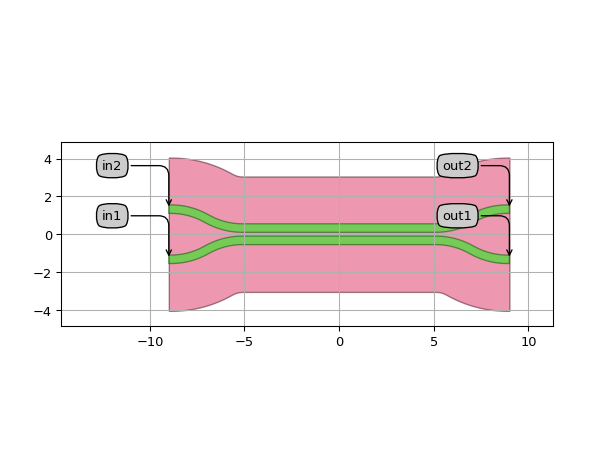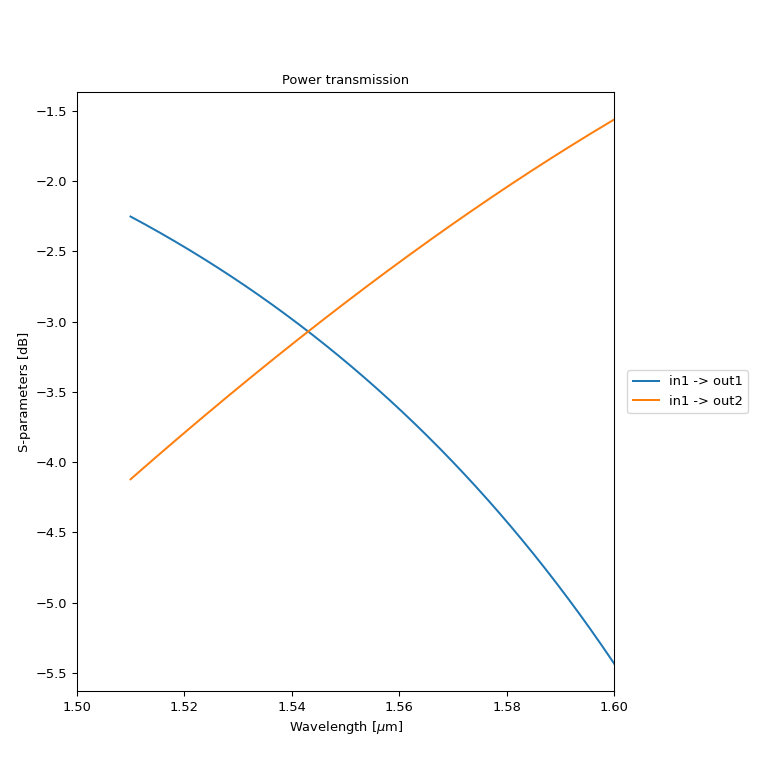## DirectionalCouplerUPower¶

This directional coupler is a PCell that uses the fitted model to obtain a specified fraction of the output power coupled into the cross arm (power_fraction) at the desired wavelength (target_wavelength). It inherits from DirectionalCouplerU, therefore the directional coupler is built using a Bezier bend as default routing method and the Caphe simulation is performed using the fitting data generated by simulating DirectionalCouplerU.

Reference

Click on the name of the component below to see the complete PCell reference.

 si_fab.all.DirectionalCouplerUPower PCell for a directional coupler that uses the fitted model to achieve a power coupling at a target wavelength

Example

from si_fab import all as pdk
import numpy as np
import pylab as plt

dc = pdk.DirectionalCouplerUPower(power_fraction=0.5,
target_wavelength=1.55)
dc_lv = dc.Layout()
dc_lv.visualize(annotate=True)

dc_cm = dc.CircuitModel()

wavelengths = np.linspace(1.51, 1.6, 500)
S = dc_cm.get_smatrix(wavelengths=wavelengths)
plt.figure()
plt.plot(wavelengths, np.abs(S["in1", "out1"])**2, linewidth=2.2, label="in1-out1 (through)")
plt.plot(wavelengths, np.abs(S["in1", "out2"])**2, linewidth=2.2, label="in1-out2 (drop)")
plt.axvline(x=dc.target_wavelength)
plt.title("Power transmission", fontsize=16)
plt.xlabel("Wavelength", fontsize=16)
plt.ylabel("Power", fontsize=16)
plt.xlim(1.5, 1.6)
plt.legend(fontsize=14, loc=1)
plt.legend()
plt.show()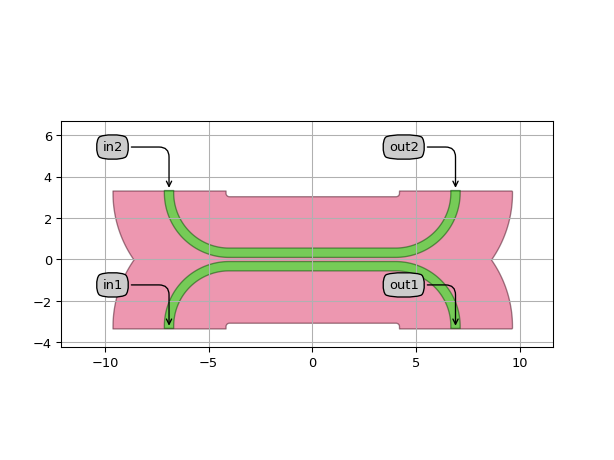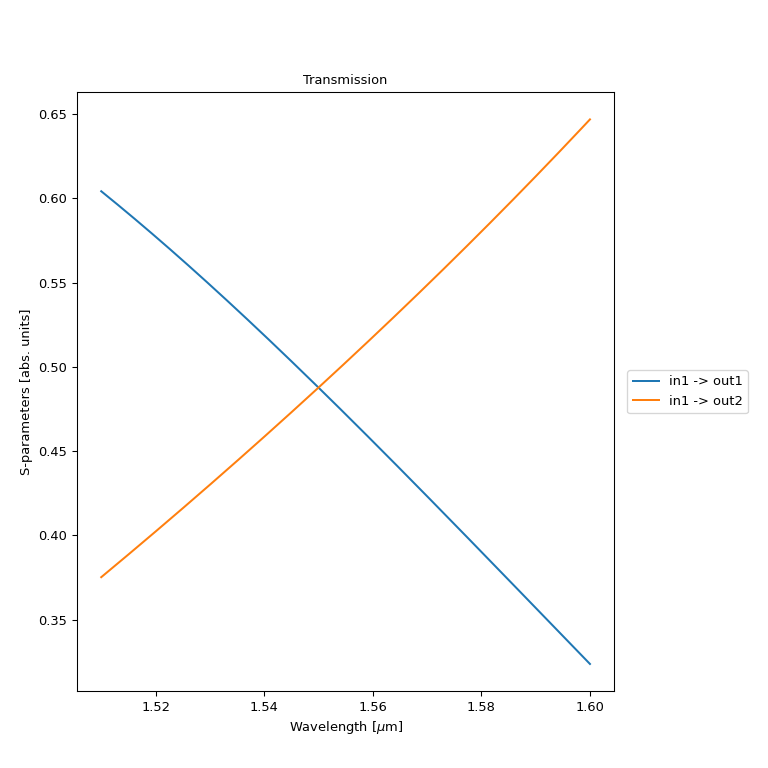## DirectionalCouplerSPower¶

This directional coupler follows the same logic as DirectionalCouplerUPower. It inherits from DirectionalCouplerS, therefore it is built using a Bezier s-bend as default routing method and the Caphe simulation is performed using the fitting data generated by simulating DirectionalCouplerS.

Reference

Click on the name of the component below to see the complete PCell reference.

 si_fab.all.DirectionalCouplerSPower PCell for a directional coupler that uses the fitted model to achieve a power coupling at a target wavelength.

Example

from si_fab import all as pdk
import numpy as np
import pylab as plt

dc = pdk.DirectionalCouplerSPower(power_fraction=0.5,
target_wavelength=1.55)
dc_lv = dc.Layout()
dc_lv.visualize(annotate=True)

dc_cm = dc.CircuitModel()

wavelengths = np.linspace(1.51, 1.6, 500)
S = dc_cm.get_smatrix(wavelengths=wavelengths)
plt.figure()
plt.plot(wavelengths, np.abs(S["in1", "out1"])**2, linewidth=2.2, label="in1-out1 (through)")
plt.plot(wavelengths, np.abs(S["in1", "out2"])**2, linewidth=2.2, label="in1-out2 (drop)")
plt.axvline(x=dc.target_wavelength)
plt.title("Power transmission", fontsize=16)
plt.xlabel("Wavelength", fontsize=16)
plt.ylabel("Power", fontsize=16)
plt.xlim(1.5, 1.6)
plt.legend(fontsize=14, loc=1)
plt.legend()
plt.show()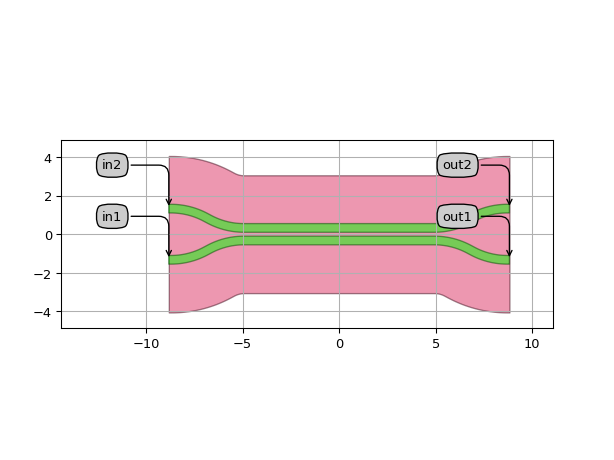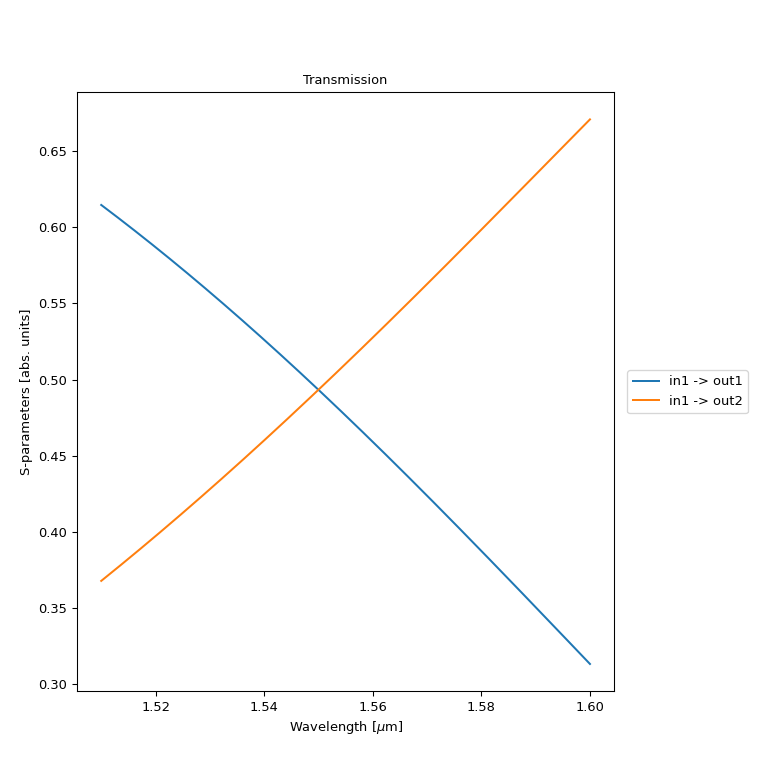## Simulation and regeneration of the data files¶

The simulations performed on DirectionalCouplerU and DirectionalCouplerS with Ansys Lumerical FDTD and with CST Studio Suite ® can be regenerated if deeper exploration of these components needs to be carried out. The regeneration can be performed using the regenerate_dc() function using the script regenerate_directional_coupler_u.py or regenerate_directional_coupler_s.py contained in si_fab/components/dir_coupler/regeneration.

The user can specify the following options:

• dir_coupler_class: the directional coupler to regenerate. The name should not contain parentheses ().
• lengths: numpy array of the lengths to be resimulated, refitted or replotted.
• wavelengths: list of wavelengths at which the simulation should be run.
• sim_sw: simulation software to be used for the simulation. The only accepted inputs are “Lumerical” and “CST”.
• resimulate: boolean. If True, the directional coupler will be simulated for all the lengths at all the wavelengths. The S-matrix results and the simulation files are stored in a smatrix.z file in data/component_name/sim_sw/lengthX.X.
• refit: boolean. If True, it performs a fitting of the data contained in the smatrix.z files for the specified lengths and at the specified wavelengths. The polynomial coefficients are stored in a params.z file in data/component_name/model. This file is loaded by Caphe to perform circuit simulations.
• plot: boolean. If True, it plots the results of the simulation and of the fitting, after these are done.

Reference

Click on the name of the functions below to see the complete API reference of the simulation and regeneration recipes.

 si_fab.components.dir_coupler.simulation.simulate_lum.simulate_dc Simulation recipe for a directional coupler in Lumerical FDTD. si_fab.components.dir_coupler.simulation.simulate_cst.simulate_dc Simulation recipe for a directional coupler in CST Studio Suite (R). si_fab.components.dir_coupler.regeneration.regen_utils.regenerate_dc Function that allows to resimulate, refit and plot the results of the directional coupler specified in dir_coupler_class.